Maths-
General
Easy

Question

# What is the co-efficient of x2y in  -14x2y

## -14714-7Hint:

## The correct answer is: -14

### The coefficient is the number which is multiplied with the algebraic expression.For example: In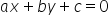the coefficient of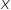is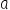and the coefficient of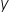is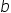So, In the given expression the coefficient of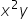is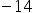.#### With Turito Foundation.#### Get an Expert Advice From Turito.